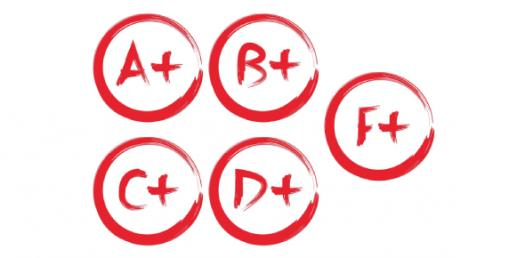# What Grade Are You In?

Approved & Edited by ProProfs Editorial Team
The editorial team at ProProfs Quizzes consists of a select group of subject experts, trivia writers, and quiz masters who have authored over 10,000 quizzes taken by more than 100 million users. This team includes our in-house seasoned quiz moderators and subject matter experts. Our editorial experts, spread across the world, are rigorously trained using our comprehensive guidelines to ensure that you receive the highest quality quizzes.
| By Garretttlovessoc
G
Garretttlovessoc
Community Contributor
Quizzes Created: 1 | Total Attempts: 13,887
Questions: 13 | Attempts: 14,419SettingsTAKE THIS QUIZ TO SEE HOW SMART YOU ARE.

• 1.

### KINDERGARTEN HOW DO YOUSPELL 1?

• A.

ONE

• B.

WON

• C.

WUN

• D.

ONF

• E.

WONE

• F.

WUNE

A. ONE
Explanation
The correct answer is "ONE" because it is the correct spelling of the number 1.

Rate this question:

• 2.

### FIRST GRADE WHAT IS 7+7?

• A.

14

• B.

13

• C.

12

• D.

15

• E.

16

• F.

17

• G.

14 AND A HALF

• H.

90

• I.

1,000,000

A. 14
Explanation
The correct answer is 14 because when you add 7 and 7 together, you get a sum of 14.

Rate this question:

• 3.

### 2ND What shape has four sides of equal length and four corners?

Square, SQUARE, square
Explanation
A line has symmetry because it can be divided into two equal halves that are mirror images of each other. This means that if you fold the line along its midpoint, both sides will match perfectly.

Rate this question:

• 4.

### 3RD WHAT IS 4X4+5=?

• A.

20

• B.

21

B. 21
Explanation
The correct answer is 21 because when you multiply 4 by 4, you get 16. Then, when you add 5 to 16, you get a total of 21.

Rate this question:

• 5.

### 6TH IS SIX PRIME OR COMPOSITE OR NIETHER?

• A.

PRIME

• B.

COMPOSITE

• C.

NIETHER

B. COMPOSITE
Explanation
The number 6 is composite because it can be divided evenly by numbers other than 1 and itself. In the case of 6, it can be divided by 2 and 3 without leaving a remainder. This means that 6 is not a prime number, which is defined as a number that can only be divided by 1 and itself. Therefore, the correct answer is composite.

Rate this question:

• 6.

### DIRIVATIVES/12TH WHAT IS THE FIRST DIRIVATIVE OF 6X+4?

• A.

6

• B.

5

A. 6
Explanation
The first derivative of 6x+4 is 6. This is because when we take the derivative of a constant term (in this case, 4), it becomes 0. The derivative of 6x is 6, as the power of x is reduced by 1 and the coefficient (6) remains the same. Therefore, the first derivative of 6x+4 is 6.

Rate this question:

• 7.

### WHAT IS THE FIRST DIRIVATIVE OF 4?

• A.

0

• B.

1

• C.

2

• D.

3

A. 0
Explanation
The first derivative of a constant value is always 0. In this case, the constant value is 4, so its first derivative will be 0. The first derivative represents the rate of change of a function at a given point, and since the function is a constant, it does not change and therefore has no rate of change.

Rate this question:

• 8.

### DOES A LINE HAVE SYMMETRY

• A.

YES

• B.

NO

• C.

MAYBE

A. YES
Explanation
A line has symmetry because it can be divided into two equal parts that are mirror images of each other.

Rate this question:

• 9.

### DOES 1+1=2

• A.

YES

• B.

NO

• C.

YES AND NO

• D.

YESNO

A. YES
Explanation
The answer is "YES" because in basic arithmetic, when you add 1 and 1 together, the result is 2.

Rate this question:

• 10.

### IS 5 PRIME

• A.

True

• B.

False

A. True
Explanation
The number 5 is considered prime because it is only divisible by 1 and itself. It does not have any other factors. Prime numbers are unique in that they cannot be formed by multiplying two smaller numbers together.

Rate this question:

• 11.

### 1=1

• A.

MAYBE YES

• B.

MAYBE NO

• C.

DEFINETLY

• D.

CAN'T SAY

C. DEFINETLY
Explanation
The answer "DEFINETLY" is the correct choice because the statement "1=1" is a mathematical equation that is always true. In mathematics, the symbol "=" denotes equality, and in this case, it means that the value of 1 is equal to the value of 1. Therefore, the answer "DEFINETLY" indicates that the statement is definitely true.

Rate this question:

• 12.

### 1+1=2.AM I WRONG?

• A.

YES

• B.

NO

• C.

MAYBE

B. NO
Explanation
The given statement "1+1=2" is a universally accepted mathematical fact. Therefore, the answer "NO" indicates that the statement is not wrong.

Rate this question:

Related TopicsBack to top